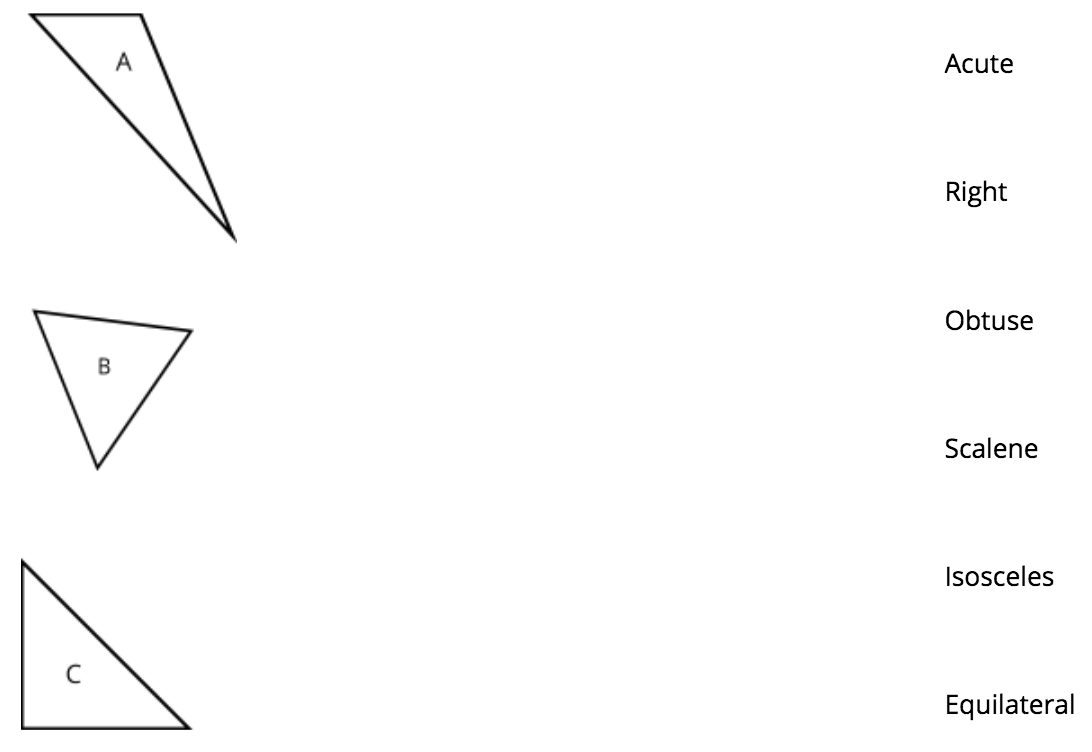# Shapes and Angles

## Objective

Analyze and classify triangles based on side length, angle measure, or both.

## Common Core Standards

### Core Standards

?

• 4.G.A.1 — Draw points, lines, line segments, rays, angles (right, acute, obtuse), and perpendicular and parallel lines. Identify these in two-dimensional figures.

• 4.G.A.2 — Classify two-dimensional figures based on the presence or absence of parallel or perpendicular lines, or the presence or absence of angles of a specified size. Recognize right triangles as a category, and identify right triangles.

?

• 3.G.A.1

## Criteria for Success

?

1. Classify triangles according to their side length, and understand each type as equilateral (has all three sides of equal length), isosceles (has two sides of equal length), and scalene (has no sides of equal length) (MP.2, MP.5).
2. Classify triangles according to their angle measure and understand each type as acute (has all three angles that are acute), right (has a right angle), and obtuse (has an obtuse angle) (MP.2, MP.5).

#### Fishtank Plus

• Problem Set
• Student Handout Editor
• Vocabulary Package

?

### Problem 1

1. Sort the shapes (cut out from Template: Triangles) into as many categories as you’d like. Then explain how you sorted them.
2. Sort the shapes (cut out from Template: Triangles) in another way. Then explain how you sorted them.

#### References

EngageNY Mathematics Grade 4 Mathematics > Module 4 > Topic D > Lesson 13Concept Development Problem 2

Grade 4 Mathematics > Module 4 > Topic D > Lesson 13 of the New York State Common Core Mathematics Curriculum from EngageNY and Great Minds. © 2015 Great Minds. Licensed by EngageNY of the New York State Education Department under the CC BY-NC-SA 3.0 US license. Accessed Dec. 2, 2016, 5:15 p.m..

Modified by Fishtank Learning, Inc.

### Problem 2

Fill out the following table based on your shapes (Template: Triangles).

 Triangle Letter Attributes (include side lengths and angle measures) Classification A B C D E F G

## Problem Set & Homework

### Discussion of Problem Set

• Which triangles in #1 are right angles? How do you know?
• Look at #2. Is scalene a way of classifying a triangle by its side lengths or angles? Can we infer anything about its classification according to angle measure? Can we infer anything about its angle measures at all?
• How do the tick marks and angle symbols allow classification of triangles without using tools in #3?
• How did you classify the triangle in #4? Explain.
• Were the statements in #5 always, sometimes, or never true? How do you know?
• Were the triangles you drew in #6 more or less difficult to classify than those in #3? Why?

?

### Problem 1

Draw a line to match each triangle below with one term that describes its angle type and one term to describe its side lengths.### Problem 2

Explain how you determined how to classify triangle C.